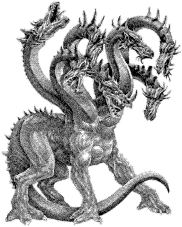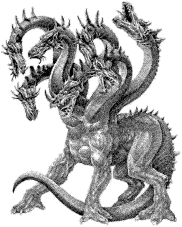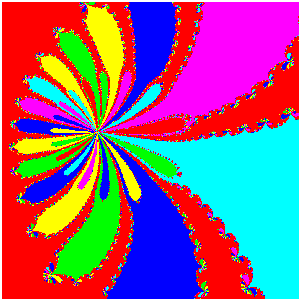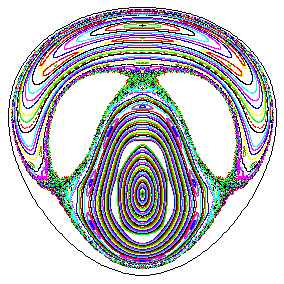HIDRA: A HOME-ASSEMBLED PARALLEL COMPUTERHIDRA is a parallel computer obtained by linking together several Pentium-based PCs, by means of a dedicated network (this is usually known as Beowulf-class computer).

The hardware used is:

• 1 PC with 2 Pentium Pro 200 processors (master node).
• 12 PCs with 2 Pentium II 266 processors each.

Every PC has 128 Mbytes of RAM and IDE hard disks of 3.2 Gbytes. They are linked together by a 100 Mbits Ethernet network (with two hubs). The master node acts as a gateway between the network of the Department and the HIDRA internal network.

We have used Linux (Slackware 3.4, kernel 2.0.33) on each node, and we use the public domain version of the PVM and MPI message passing libraries for the communications.

The initial benchmarks show a sustained performance of 2 Gigaflop in several applications we are interested in (simulations of some dynamical systems), but it seems that it could deliver 3 times this performance using very specific (and useless!) benchmarks.

The Barcelona UB-UPC Dynamical Systems Group works, among other things, on several projects that require a huge amount of computations (i.e., numerical simulations of continuous and discrete systems, bifurcation analysis, numeric and symbolic computation of invariant manifolds, etc.). In this context, HIDRA is a very good solution for our computing necessities since many of the algorithms we use can be parallelized very efficiently.

The computing needs of the Group

Most of the research projects involve Dynamical Systems (discrete and continuous, parametric studies, bifurcation analysis, computation of invariant manifolds, etc, both numerically and symbolically). Symbolic computations with ad hoc manipulators including up to several millions of terms and arbitrary precision long tasks are also done. Typically a project can require up to 10^15 elementary arithmetic operations. Systems of low dimension (e.g., the number of variables and parameters is at most 6 or 7) are analyzed exhaustively to obtain a description of the phase space as complete as possible. Higher dimensions are only analyzed partially. All the related software is developed, analyzed and implemented by members of the Group.

A Beowulf cluster seems to be a good solution to satisfy these needs. It consists of

• A pile of PC,
• A dedicated network connecting them,
• A communications software
• A software allowing to start/stop processes and to pass data from one processor to another.
This is the basic configuration of HIDRA, as described above.

Programming tasks for this parallel machine is easy. The conversion from a program running on a sequential computer to a parallel one is simple if the program is structured as a main code calling many times some computing routine and avoiding unnecessary data transmission. Anyway an effort is required to investigate if alternative algorithm, may be not efficient on a sequential computer, can have a higher performance on a parallel one. In any case, to have a good performance, a detailed analysis and comparison of algorithms is essential.

A presentation demo is described below. All times refer to "clock" times, as estimated manually. Almost all of them have an important part of graphics, in contrasts with the usual tasks of the Group, where graphics play a role for presentation just at the end of a long computation. No effort has been made to optimize graphic routines.

1. The unavoidable computation of the Mandelbrot set for quadratic complex maps. A test using 1600 x 1200 pixels in the black region of the set, where computations are stopped after 2560 iterates, takes 25 seconds. The number of operations for iteration (including tests!) is 10. This gives an average performance close to 2 Gflop/s.

Click on the figure to see a sequence of 6 magnifications with resolution 800x800 pixels each. The first one is 35K large.2. Computation of the prime numbers between 1 and 2^31-1 using a Fortran program. A sieve method is used. Typically a variable for each number is set to 1, then the multiples of primes 2,3,5,...,46337 (larger than those primes) are set to 0. Finally the variables are examined to see the ones which remain equal to 1. These are the primes. The cost is: a) to put to 1, b) to cancel (the sum of the inverses of the above primes is close to 2.64), c) to scan the list. Every step requires to move an index and to set or look for a number. The total estimated cost is 2(2+2.64)(2^31-1)=19.93 Giga. The clock time for the run is 10 seconds.

Click here to see two figures about prime number density.

3. Computation of basins of attraction for the Newton method when looking for the roots of a (complex) polynomial in a domain of the complex plane. Each pixel is set to a color depending on the root to which it converges. All computation are done in real*8 variables. If the degree of the polynomial is n, the computation cost of each Newton iterate an scan for the proximity to one of the roots is 16(n+1). For the program use is made of the knowledge of the roots (computed at the beginning) to speed up the task. The total cost depends strongly of the polynomial and on the domain. A run for a degree 32 polynomial on a suitable square domain of size 10^(-10) around a preimage of a critical point requires in total 1.124E8 iterates of Newton method. The computing (and displaying) time is of 27 seconds, giving an average performance of 2.2 Gflop/s.

Click on the figure for a 800x800 magnification (39K).4. Computation of the phase portrait on a Poincaré section of the classical Hénon-Heiles cubic potential in two degrees of freedom on the level of energy h=0.12. For each of 250 initial points 4000 iterates have been computed. A Taylor method of order 24, with derivatives obtained by recurrent use of Leibnitz method has shown to be very efficient. The effort of each integration step is, roughly, 3000 operations. A total of 4.6E9 steps is required and the total computing time is 23 seconds. The accuracy of the integration is measured by the maximal change of the energy for initial energies in (0,1/6) and a scan of the Poincaré section for the initial point. In all cases it is below 2E-16. Note that the "classical" order 4 Runge-Kutta method gives, in the best case, an error 30 times larger with a computing time also 30 times larger. Computations with the same cost display errors of 3E-9. Even the better RKF78 method is only able to reduce the figure 30 to 3 in both places and with the same cost produces error up to 3E-11. Here we attain 2 Gflop/s.

Click on the figure for a 784x784 magnification (105K).5. Computation of the rotation number for the quadratic Hénon conservative area preserving map for different initial values of the distance to the elliptic fixed point and different values of the parameter. The rotation number is simply estimated by "counting revolutions" (of course, we use typically more efficient methods, but this is a demo!). Each iterate requires 10 operations (including tests for revolution and eventual escape). A run with 100 values of the parameter, 400 distances for every value of the parameter and 100,000 iterates (provided they do not escape) per point requires, in total, 2.7E10 iterates. It takes a computing time of 13.5 seconds, with an average performance of 2 Gflop/s.

6. The motion around one of the collinear points of the spatial circular restricted three-body problem is unstable. However this is a nice place for spacecraft missions. Several missions have already been launched (ISEE-3 and SOHO). Many other are planned. Their orbits lie on the "center manifold" of the collinear points. This manifold can be approximated by symbolic computation to any desired order, and the Hamiltonian can be reduced to this manifold, skipping in this way the instability in the computations. This reduction has been performed by a previous program (up to order 46) and the demo just takes the degree 6 approximation of the Hamiltonian. It contains 55 terms. A computation with 75 initial points and 1000 iterates per point, on a suitable Poincaré section takes 53 seconds.

Click on the figure for a 850x680 magnification (45K).7. A more realistic computation is done using the full solar system (i.e. Sun, planets and Moon) to study the stability zones around the triangular libration points of the Earth-Moon system. JPL ephemeris are used and this requires the computation of the positions of all these bodies by a Chebyshev interpolation polynomial on a suitable time interval (which changes from body to body). Then Newton equations of motion are integrated, to determine the evolution of a massless particle, by using a RKF78 algorithm. The average global number of integration steps delivered by HIDRA is 30,000 steps/second.
Summary

HIDRA is a very efficient tool for most of the computing tasks we undertake.

Difficulties: It can require an effort to obtain the most suitable algorithms to improve the performance. Problems in high dimension (e.g., numerical solution of partial differential equations) would benefit from shared memory or a much faster communication.

Advantages: Beyond the know how we have developed, this is a tool to solve problems and, also, a source of theoretical and practical problems. From another point of view is fully scalable, updatable and recyclable.

Future: It is planned to increase the number of nodes to 24, to increase the amount of RAM memory of each PC, to update gradually the nodes to faster processors, depending on the best available performance/price, and to increase the communications hardware if this shows to be a bottle neck for some problems.

Acknowledgments

The construction of HIDRA has been made possible thanks to grants of the Spanish Ministry of Culture and Education, the Generalitat de Catalunya and the support of the University of Barcelona.

We specially want to thank the people around the Linux, GNU and PVM projects, for putting in the public domain such a good software. Without them, this would have been only a dream (or a nightmare? :-)).

Barcelona, march 25th 1998.

Joaquim Font, Àngel Jorba, Carles Simó, Jaume Timoneda (click here for a picture)Back to the UB-UPC DSG home page